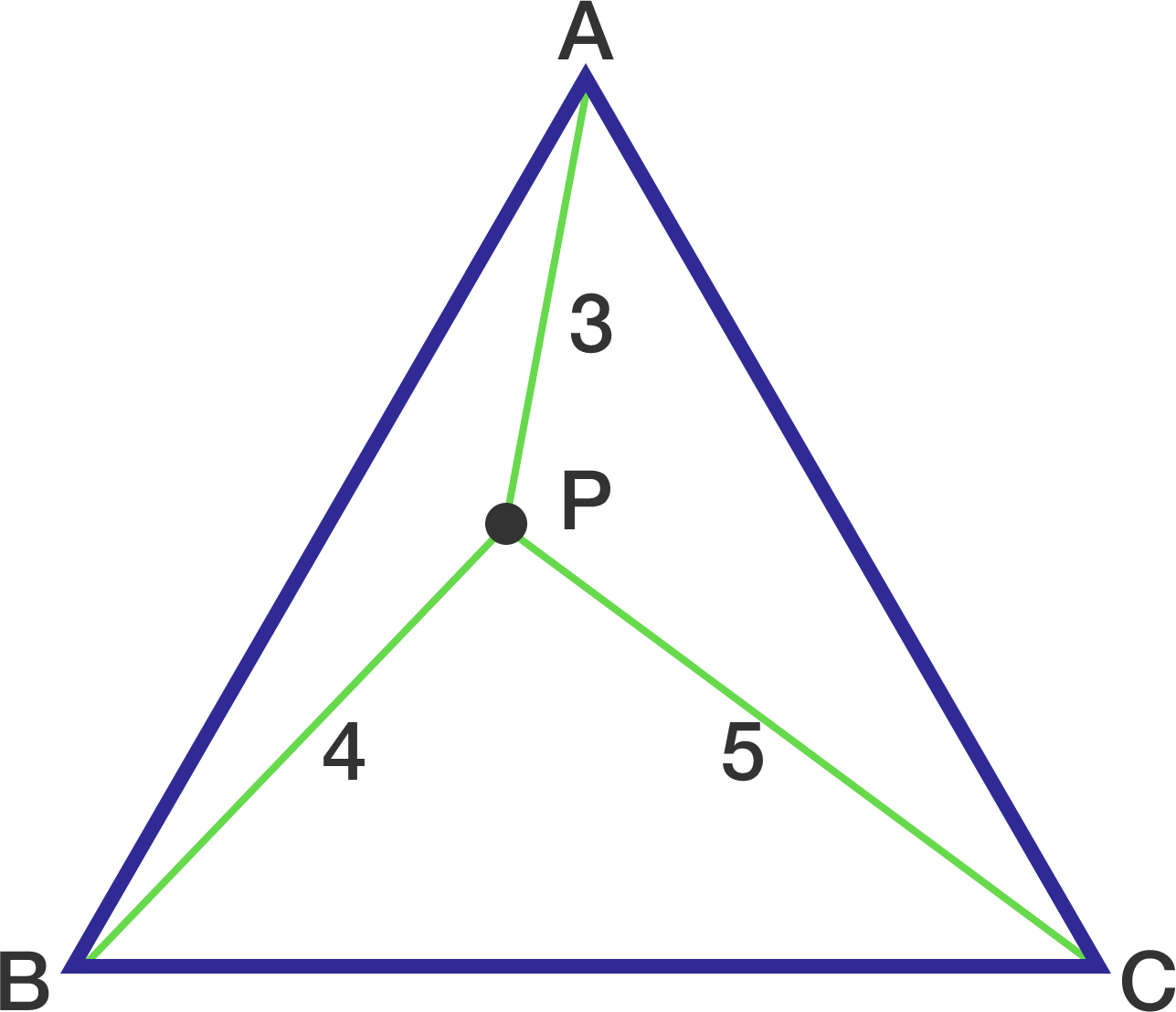# Area without the required lengths.

Geometry Level 3

Let $P$ be a point inside an equilateral triangle $ABC$ such that $PA = 3, PB =4, PC =5.$ Find the area of $\triangle ABC$ to 2 decimal places.×

Problem Loading...

Note Loading...

Set Loading...